At SAS Global Forum 2019, Daymond Ling presented an interesting discussion of binary classifiers in the financial industry. The discussion is motivated by a practical question: If you deploy a predictive model, how can you assess whether the model is no longer working well and needs to be replaced?

Daymond discussed the following three criteria for choosing a model:

1. Discrimination: The ability of the binary classifier to predict the class of a labeled observation. The area under an ROC curve is one measure of a binary model's discrimination power. In SAS, you can compute the ROC curve for any predictive model.
2. Accuracy: The ability of the model to estimate the probability of an event. The calibration curve is a graphical indication of a model's accuracy. In SAS, you can compute a calibration curve manually, or you can use PROC LOGISTIC in SAS/STAT 15.1 to automatically compute a calibration curve.
3. Stability: Point estimates are often used to choose a model, but you should be aware of the variability of the estimates. This is a basic concept in statistics: When choosing between two unbiased estimators, you should usually choose the one that has smaller variance. SAS procedures provide (asymptotic) standard errors for many statistics such as the area under an ROC curve. If you have reason to doubt the accuracy of an asymptotic estimate, you can use bootstrap methods in SAS to estimate the sampling distribution of the statistic.

### Estimates of model stability

My article about comparing the ROC curves for predictive models contains two competing models: A model from using PROC LOGISTIC and an "Expert model" that was constructed by asking domain experts for their opinions. (The source of the models is irrelevant; you can use any binary classifier.) You can download the SAS program that produces the following table, which estimates the area under each ROC curve, the standard error, and 90% confidence intervals: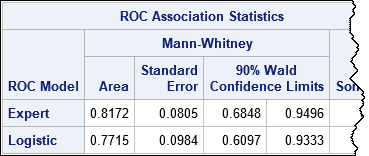The "Expert" model has a larger Area statistic and a smaller standard error, so you might choose to deploy it as a "champion model."

In his presentation, Daymond asked an important question. Suppose one month later you run the model on a new batch of labeled data and discover that the area under the ROC curve for the new data is only 0.73. Should you be concerned? Does this indicate that the model has degraded and is no longer suitable? Should you cast out this model, re-train all the models (at considerable time and expense), and deploy a new "champion"?

The answer depends on whether you think Area = 0.73 represents a degraded model or whether it can be attributed to sampling variability. The statistic 0.73 is barely more than 1 standard error away from the point estimate, and you will recall that 68% of a normal distribution is within one standard deviation of the mean. From that point of view, the value 0.73 is not surprising. Furthermore, the 90% confidence interval indicates that if you run this model every day for 100 days, you will probably encounter statistics lower than 0.68 merely due to sampling variability. In other words, a solitary low score might not indicate that the model is no longer valid.

### Bootstrap estimates of model stability

If "asymptotic normality" makes you nervous, you can use the bootstrap method to obtain estimates of the standard error and the distribution of the Area statistic. The following table summarizes the results of 5,000 bootstrap replications. The results are very close to the asymptotic results in the previous table. In particular, the standard error of the Area statistic is estimated as 0.08 and in 90% of the bootstrap samples, the Area was in the interval [0.676, 0.983]. The conclusion from the bootstrap computation is the same as for the asymptotic estimates: you should expect the Area statistic to bounce around. A value such as 0.73 is not unusual and does not necessarily indicate that the model has degraded.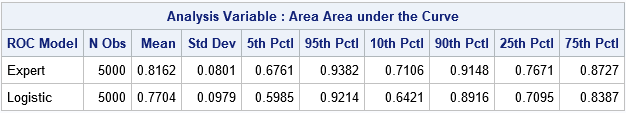You can use the bootstrap computations to graphically reveal the stability of the two models. The following comparative histogram shows the bootstrap distributions of the Area statistic for the "Expert" and "Logistic" models. You can see that not only is the upper distribution shifted to the right, but it has less variance and therefore greater stability.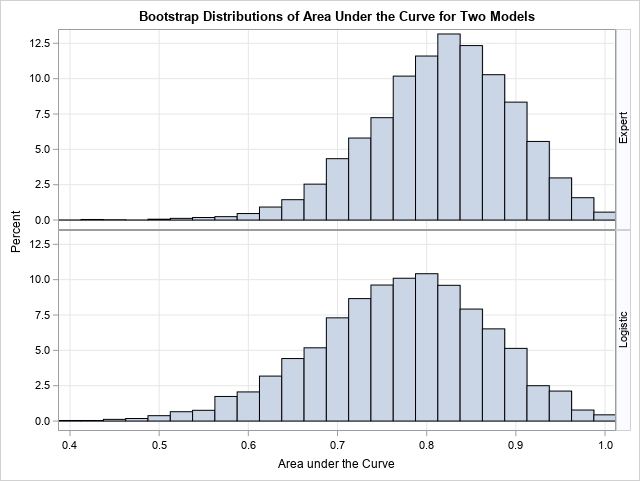I think Daymond's main points are important to remember. Namely, discrimination and accuracy are important for choosing a model, but understanding the stability of the model (the variation of the estimates) is essential for determining when a model is no longer working well and should be replaced. There is no need to replace a model for a "bad score" if that score is within the range of typical statistical variation.

### References

Ling, D. (2019), "Measuring Model Stability", Proceedings of the SAS Global Forum 2019 Conference.

The post Discrimination, accuracy, and stability in binary classifiers appeared first on The DO Loop.At SAS Global Forum 2019, Daymond Ling presented an interesting discussion of binary classifiers in the financial industry. The discussion is motivated by a practical question: If you deploy a predictive model, how can you assess whether the model is no longer working well and needs to be replaced?

Daymond discussed the following three criteria for choosing a model:

1. Discrimination: The ability of the binary classifier to predict the class of a labeled observation. The area under an ROC curve is one measure of a binary model's discrimination power. In SAS, you can compute the ROC curve for any predictive model.
2. Accuracy: The ability of the model to estimate the probability of an event. The calibration curve is a graphical indication of a model's accuracy. In SAS, you can compute a calibration curve manually, or you can use PROC LOGISTIC in SAS/STAT 15.1 to automatically compute a calibration curve.
3. Stability: Point estimates are often used to choose a model, but you should be aware of the variability of the estimates. This is a basic concept in statistics: When choosing between two unbiased estimators, you should usually choose the one that has smaller variance. SAS procedures provide (asymptotic) standard errors for many statistics such as the area under an ROC curve. If you have reason to doubt the accuracy of an asymptotic estimate, you can use bootstrap methods in SAS to estimate the sampling distribution of the statistic.

### Estimates of model stability

My article about comparing the ROC curves for predictive models contains two competing models: A model from using PROC LOGISTIC and an "Expert model" that was constructed by asking domain experts for their opinions. (The source of the models is irrelevant; you can use any binary classifier.) You can download the SAS program that produces the following table, which estimates the area under each ROC curve, the standard error, and 90% confidence intervals:The "Expert" model has a larger Area statistic and a smaller standard error, so you might choose to deploy it as a "champion model."

In his presentation, Daymond asked an important question. Suppose one month later you run the model on a new batch of labeled data and discover that the area under the ROC curve for the new data is only 0.73. Should you be concerned? Does this indicate that the model has degraded and is no longer suitable? Should you cast out this model, re-train all the models (at considerable time and expense), and deploy a new "champion"?

The answer depends on whether you think Area = 0.73 represents a degraded model or whether it can be attributed to sampling variability. The statistic 0.73 is barely more than 1 standard error away from the point estimate, and you will recall that 68% of a normal distribution is within one standard deviation of the mean. From that point of view, the value 0.73 is not surprising. Furthermore, the 90% confidence interval indicates that if you run this model every day for 100 days, you will probably encounter statistics lower than 0.68 merely due to sampling variability. In other words, a solitary low score might not indicate that the model is no longer valid.

### Bootstrap estimates of model stability

If "asymptotic normality" makes you nervous, you can use the bootstrap method to obtain estimates of the standard error and the distribution of the Area statistic. The following table summarizes the results of 5,000 bootstrap replications. The results are very close to the asymptotic results in the previous table. In particular, the standard error of the Area statistic is estimated as 0.08 and in 90% of the bootstrap samples, the Area was in the interval [0.676, 0.983]. The conclusion from the bootstrap computation is the same as for the asymptotic estimates: you should expect the Area statistic to bounce around. A value such as 0.73 is not unusual and does not necessarily indicate that the model has degraded.You can use the bootstrap computations to graphically reveal the stability of the two models. The following comparative histogram shows the bootstrap distributions of the Area statistic for the "Expert" and "Logistic" models. You can see that not only is the upper distribution shifted to the right, but it has less variance and therefore greater stability.I think Daymond's main points are important to remember. Namely, discrimination and accuracy are important for choosing a model, but understanding the stability of the model (the variation of the estimates) is essential for determining when a model is no longer working well and should be replaced. There is no need to replace a model for a "bad score" if that score is within the range of typical statistical variation.

### References

Ling, D. (2019), "Measuring Model Stability", Proceedings of the SAS Global Forum 2019 Conference.

The post Discrimination, accuracy, and stability in binary classifiers appeared first on The DO Loop.Many SAS procedures support the BY statement, which enables you to perform an analysis for subgroups of the data set. Although the SAS/IML language does not have a built-in "BY statement," there are various techniques that enable you to perform a BY-group analysis. The two I use most often are the UNIQUE-LOC technique and the UNIQUEBY technique. The first is more intuitive, the second is more efficient. This article shows how to use SAS/IML to read and process BY-group data from a data set.

I previously showed that you can perform BY-group processing in SAS/IML by using the UNIQUEBY technique, so this article uses the UNIQUE-LOC technique. The statistical application is simulating clusters of data. If you have a SAS data set that contains the centers and covariance matrices for several groups of observations, you can then read that information into SAS/IML and simulate new observations for each group by using a multivariate normal distribution.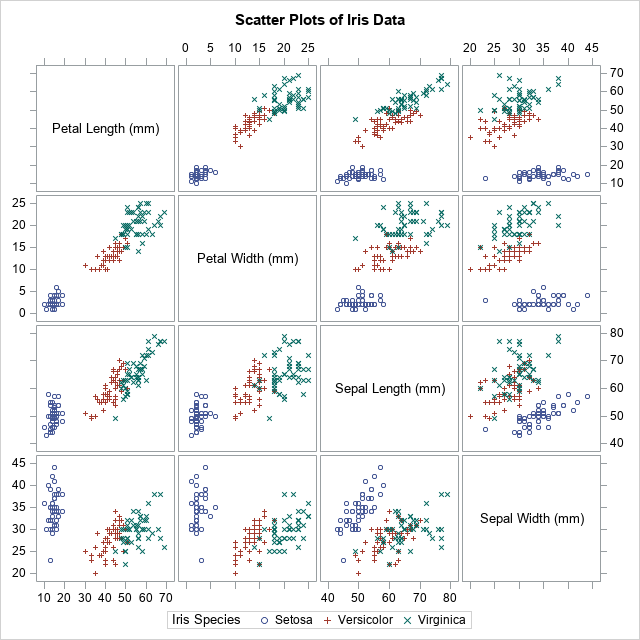### Matrix operations and BY groups

A BY-group analysis in SAS/IML usually starts with a SAS data set that contains a bunch of covariance or correlation matrices. A simple example is a correlation analysis of each species of flower in Fisher's iris data set. The BY-group variable is the species of iris: Setosa, Versicolor, or Virginica. The variables are measurements (in mm) of the sepals and petals of 150 flowers, 50 from each species. A panel of scatter plots for the iris data is shown to the right. You can see that the three species appear to be clustered. From the shapes of the clusters, you might decide to model each cluster by using a multivariate normal distribution.

You can use the OUTP= and COV options in PROC CORR to output mean and covariance statistics for each group, as follows:

```proc corr data=sashelp.iris outp=CorrOut COV noprint; by Species; var Petal: Sepal:; run;   proc print data=CorrOut(where=(_TYPE_ in ("N", "MEAN", "COV"))) noobs; where Species="Setosa"; /* just view information for one group */ by Species _Type_ notsorted; var _NAME_ Petal: Sepal:; run;```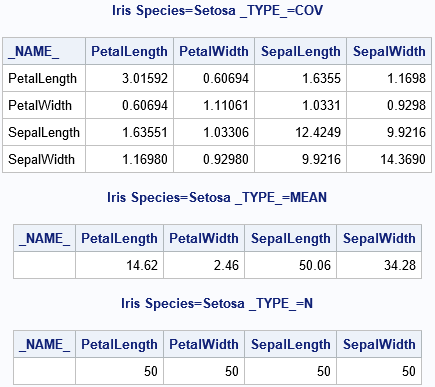The statistics for one of the groups (Species='Setosa') are shown. The number of observations in the group (N) is actually a scalar value, but it was replicated to fit into a rectangular data set.

### Reading BY-group information into SAS/IML

This section reads the sample size, mean vector, and covariance matrix for all groups. A WHERE clause selects only the observations of interest:

```/* Read in N, Mean, and Cov for each species. Use to create a parametric bootstrap by simulating N[i] observations from a MVN(Mean[i], Cov[i]) distribution */ proc iml; varNames = {'PetalLength' 'PetalWidth' 'SepalLength' 'SepalWidth'}; use CorrOut where (_TYPE_="N" & Species^=" "); /* N */ read all var varNames into mN[rowname=Species]; /* read for all groups */ print mN[c=varNames];   use CorrOut where (_TYPE_="MEAN" & Species^=" "); /* mean */ read all var varNames into mMean[rowname=Species]; /* read for all groups */ print mMean[c=varNames];   use CorrOut where (_TYPE_="COV" & Species^=" "); /* covariance */ read all var varNames into mCov[rowname=Species]; /* read for all groups */ close; print mCov[c=varNames];```

The output (not shown) shows that the matrices mN, mMean, and mCov contain the vertical concatenation (for all groups) of the sample size, mean vectors, and covariance matrices, respectively.

The grouping variable is Species. You can use the UNIQUE function to get the unique (sorted) values of the groups. You can then iterate over the unique values and use the LOC function to extract only the rows of the matrices that correspond to the ith group. What you do with that information depends on your application. In the following program, the information for each group is used to create a random sample from a multivariate normal distribution that has the same size, mean, and covariance as the ith group:

```/* Goal: Write random MVN values in X to data set */ X = j(1, ncol(varNames), .); /* X variables will be numeric */ Spec = BlankStr(nleng(Species)); /* and Spec will be character */ create SimOut from X[rowname=Spec colname=varNames]; /* open for writing */   /* The BY-group variable is Species */ call randseed(12345); u = unique(Species); /* get unique values for groups */ do i = 1 to ncol(u); /* iterate over unique values */ idx = loc(Species=u[i]); /* rows for the i_th group */ N = mN[i, 1]; /* extract scalar from i_th group */ mu = mMean[i,]; /* extract vector from i_th group */ Sigma = mCov[idx,]; /* extract matrix from i_th group */ /* The parameters for this group are now in N, mu, and Sigma. Do something with these values. */ X = RandNormal(N, mu, Sigma); /* simulate obs for the i_th group */ X = round(X); /* measure to nearest mm */ Spec = j(N, 1, u[i]); /* ID for this sample */ append from X[rowname=Spec]; end; close SimOut; quit;   ods graphics / attrpriority=none; title "Parametric Bootstrap Simulation of Iris Data"; proc sgscatter data=SimOut(rename=(Spec=Species)); matrix Petal: Sepal: / group=Species; run;```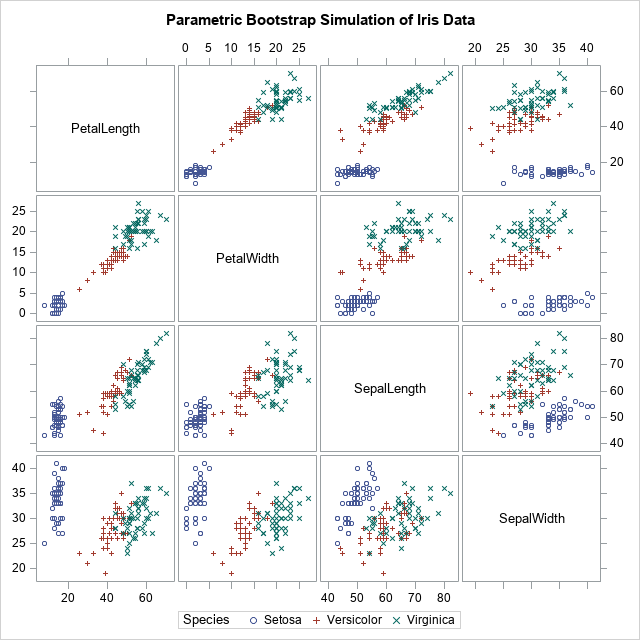The simulation has generated a new set of clustered data. If you compare the simulated data with the original, you will notice many statistical similarities.

Although the main purpose of this article is to discuss BY-group processing in SAS/IML, I want to point out that the simulation in this article is an example of the parametric bootstrap. Simulating Data with SAS (Wicklin, 2013) states that "the parametric bootstrap is nothing more than the process of fitting a model distribution to the data and simulating data from the fitted model." That is what happens in this program. The sample means and covariance matrices are used as parameters to generate new synthetic observations. Thus, the parametric bootstrap technique is really a form of simulation where the parameters for the simulation are obtained from the data.

In conclusion, sometimes you have many matrices in a SAS data set, each identified by a categorical variable. You can perform "BY-group processing" in SAS/IML by reading in all the matrices into a big matrix and then use the UNIQUE-LOC technique to iterate over each matrix.

The post Matrix operations and BY groups appeared first on The DO Loop.When I run a bootstrap analysis, I create graphs to visualize the distribution of the bootstrap statistics. For example, in my article about how to bootstrap the difference of means in a two-sample t test, I included a histogram of the bootstrap distribution and added reference lines to indicate a confidence interval for the difference of means.

For t tests, the TTEST procedure supports the BOOTSTRAP statement, which automates the bootstrap process for standard one- and two-sample t tests. A new feature in SAS/STAT 15.1 (SAS 9.4M6) is that the TTEST procedure supports the PLOTS=BOOTSTRAP option, which automatically creates histograms, Q-Q plots, and scatter plots of various bootstrap distributions.

To demonstrate the new PLOTS=BOOTSTRAP option, I will use the same example that I used to demonstrate the BOOTSTRAP statement. The data are the sedans and SUVs in the Sashelp.Cars data. The research question is to estimate the difference in fuel efficiency, as measured by miles per gallon during city driving. The bootstrap analysis enables you to visualize the approximate sampling distribution of the difference-of-mean statistic and its standard deviation. The following statements create the data and run PROC TTEST to generate the analysis. The PLOTS=BOOTSTRAP option generates the bootstrap graphs. The BOOTSTRAP statement request 5,000 bootstrap resamples and 95% confidence intervals, based on the percentiles of the bootstrap distribution:

```/* create data set that has two categories: 'Sedan' and 'SUV' */ data Sample; set Sashelp.Cars(keep=Type MPG_City); if Type in ('Sedan' 'SUV'); run;   ods trace on; title "Bootstrap Estimates with Percentile CI"; proc ttest data=Sample plots=bootstrap; class Type; var MPG_City; bootstrap / seed=123 nsamples=5000 bootci=percentile; run;```

The TTEST procedure creates seven tables and eight graphs. The previous article displayed and discussed several tables, so here I display only two of the graphs.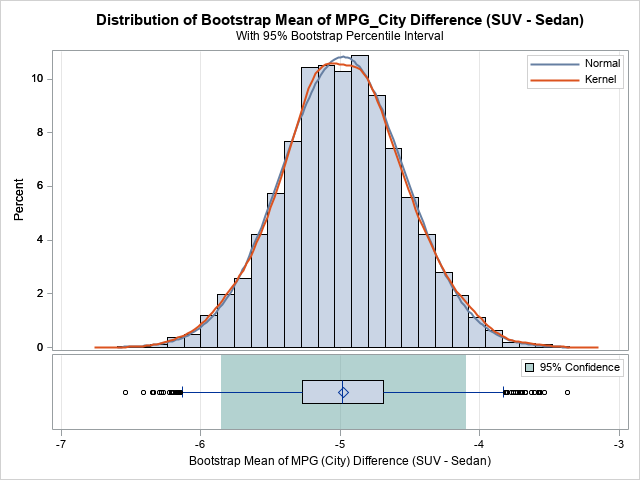The first graph is a histogram of the bootstrap distribution of the difference between the sample means for each vehicle type. The distribution appears to be symmetric and approximately normal. The middle of the distribution is close to -5, which is the point estimate for the difference in MPG_City between the SUVs and the sedans in the data. How much should we trust that point estimate? The green region indicates that 95% of the bootstrap samples had differences that were in the green area underneath the histogram, which is approximately [-5.9, -4.1]. This is a 95% confidence interval for the difference.

Similar histograms (not shown) are displayed for two other statistics: the pooled standard deviation (of the difference between means) and the Satterthwaite standard deviation. The procedure also creates Q-Q plots of the bootstrap distributions.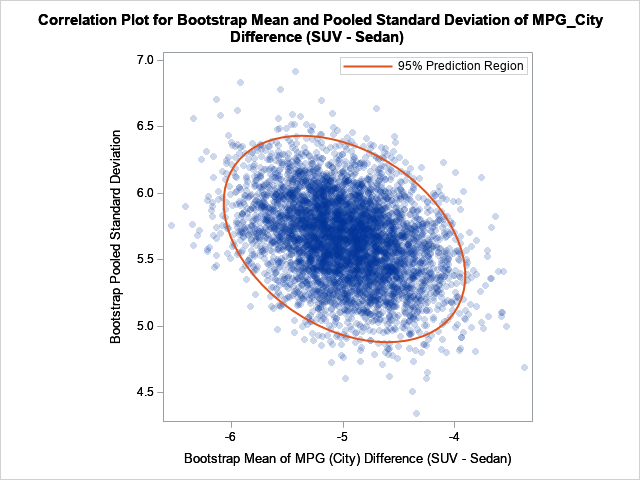The second graph is a scatter plot that shows the joint bootstrap distribution of the mean difference and the pooled standard deviations of the difference, based on 5,000 bootstrap samples. You can see that the statistics are slightly correlated. A sample that has a large (absolute) mean difference also tends to have a relatively large standard deviation. The 95% prediction region for the joint distribution indicates how these two statistics co-vary among random samples.

In summary, the TTEST procedure in SAS/STAT 15.1 supports a new PLOTS=BOOTSTRAP option, which automatically creates many graphs that help you to visualize the bootstrap distributions of the statistics. If you are conducting a bootstrap analysis for a t test, I highly recommend using the plots to visualize the bootstrap distributions and results

The post Graphs of bootstrap statistics in PROC TTEST appeared first on The DO Loop.This article describes best practices and techniques that every data analyst should know before bootstrapping in SAS. The bootstrap method is a powerful statistical technique, but it can be a challenge to implement it efficiently. An inefficient bootstrap program can take hours to run, whereas a well-written program can give you an answer in an instant. If you prefer "instants" to "hours," this article is for you! I’ve compiled dozens of resources that explain how to compute bootstrap statistics in SAS.

## Overview: What is the bootstrap method?

Recall that a bootstrap analysis enables you to investigate the sampling variability of a statistic without making any distributional assumptions about the population. For example, if you compute the skewness of a univariate sample, you get an estimate for the skewness of the population. You might want to know the range of skewness values that you might observe from a second sample (of the same size) from the population. If the range is large, the original estimate is imprecise. If the range is small, the original estimate is precise. Bootstrapping enables you to estimate the range by using only the observed data.

In general, the basic bootstrap method consists of four steps:

1. Compute a statistic for the original data.
2. Use the DATA step or PROC SURVEYSELECT to resample (with replacement) B times from the data. The resampling process should respect the null hypothesis or reflect the original sampling scheme. For efficiency, you should put all B random bootstrap samples into a single data set.
3. Use BY-group processing to compute the statistic of interest on each bootstrap sample. The BY-group approach is much faster than using macro loops. The union of the statistic is the bootstrap distribution, which approximates the sampling distribution of the statistic under the null hypothesis. Don't forget to turn off ODS when you run BY-group processing!
4. Use the bootstrap distribution to obtain estimates for the bias and standard error of the statistic and confidence intervals for parameters.

The links in the previous list provide examples of best practices for bootstrapping in SAS. In particular, do not fall into the trap of using a macro loop to "resample, analyze, and append." You will eventually get the correct bootstrap estimates, but you might wait a long time to get them!

• Programming: You can write a SAS DATA step program or a SAS/IML program that resamples from the data and analyzes each (re)sample. The programming approach gives you complete control over all aspects of the bootstrap analysis.
• Macros: You can use the %BOOT and %BOOTCI macros that are supplied by SAS. The macros handle a wide variety of common bootstrap analyses.
• Procedures: You can use bootstrap options that are built into several SAS procedures. The procedure internally implements the bootstrap method for a particular set of statistics.

## Programming the basic bootstrap in SAS

The articles in this section describe how to program the bootstrap method in SAS for basic univariate analyses, for regression analyses, and for related resampling techniques such as the jackknife and permutation tests. This section also links to articles that describe how to generate bootstrap samples in SAS.

### Examples of basic bootstrap analyses in SAS

• The basic bootstrap in SAS: SAS enables you to resample the data by using PROC SURVEYSELECT. When coupled with BY-group processing, you can perform a very efficient bootstrap analysis in SAS, including the estimate of standard errors and percentile-based confidence intervals.
• The basic bootstrap in SAS/IML: The SAS/IML language provides a compact language for bootstrapping, as shown in this basic bootstrap example.
• The smooth bootstrap: As originally conceived, a bootstrap sample contains replicates of the data. However, there are situations when "jittering" the data provides a better approximation of the sampling distribution.
• Bias-corrected and adjusted (BCa) confidence interval: For highly skewed data, the percentile-based confidence intervals are less efficient than the BCa confidence interval.
• Bootstrap the difference of means between two groups: This example shows how to bootstrap a statistic in a two-sample t test.

### Examples of bootstrapping for regression statistics

When you bootstrap regression statistics, you have two choices for generating the bootstrap samples:

• Case resampling: You can resample the observations (cases) to obtain bootstrap samples of the responses and the explanatory variables.
• Residual resampling: Alternatively, you can bootstrap regression parameters by fitting a model and resampling from the residuals to obtain new responses.

### Jackknife and permutation tests in SAS

• The jackknife method: The jackknife in an alternative nonparametric method for obtaining standard errors for statistics. It is deterministic because it uses leave-one-out samples rather than random samples.
• Permutation tests: A permutation test is a resampling technique that is closely related to the bootstrap. You permute the observations between two groups to test whether the groups are significantly different.

### Generate bootstrap sampling

An important part of a bootstrapping is generating multiple bootstrap samples from the data. In SAS, there are many ways to obtain the bootstrap samples:

• Sample with replacement: The most common resampling technique is to randomly sample with replacement from the data. You can use the SAS DATA step, the SURVEYSELECT procedure, or the SAMPLE function in SAS/IML.
• Samples in random order: It is sometimes useful to generate random samples in which the order of the observations is randomly permuted.
• Balanced bootstrap resampling: Instead of random samples, some experts advocate a resampling algorithm in which each observation appears exactly B times in the union of the B bootstrap samples.

## Bootstrap macros in SAS

The SAS-supplied macros %BOOT, %JACK, and %BOOTCI, can perform basic bootstrap analyses and jackknife analyses. However, they require a familiarity with writing and using SAS macros. If you are interested, I wrote an example that shows how to use the %BOOT and %BOOTCI macros for bootstrapping. The documentation also provides several examples.

## SAS procedures that support bootstrapping

Many SAS procedures not only compute statistics but also provide standard errors or confidence intervals that enable you to infer whether an estimate is precise. Many confidence intervals are based on distributional assumptions about the population. ("If the errors are normally distributed, then....") However, the following SAS procedures provide an easy way to obtain a distribution-free confidence interval by using the bootstrap. See the SAS/STAT documentation for the syntax for each procedure.

• PROC CAUSALMED introduced the BOOTSTRAP statement in SAS/STAT 14.3 (SAS 9.4M5). The statement enables you to compute bootstrap estimates of standard errors and confidence intervals for various effects and percentages of total effects.
• PROC MULTTEST supports the BOOTSTRAP and PERMUTATION options, which enable you to compute estimates of p-values that make no distributional assumptions.
• PROC NLIN supports the BOOTSTRAP statement, which computes bootstrap confidence intervals for parameters and bootstrap estimates of the covariance of the parameter estimates.
• PROC QUANTREG supports the CI=RESAMPLING option to construct confidence intervals for regression quantiles.
• The SURVEYMEANS, SURVEYREG, SURVEYLOGISTIC, SURVEYPHREG, SURVEYIMPUTE and SURVEYFREQ procedures introduced the VARMETHOD=BOOTSTRAP option SAS 9.4M5. The option enables you to compute bootstrap estimates of variance. With the exception of SURVEYIMPUTE, these procedures also support jackknife estimates. The jackknife is similar to the bootstrap but uses a leave-one-out deterministic scheme rather than random resampling.
• PROC TTEST introduced the BOOTSTRAP statement in SAS/STAT 14.3. The statement enables you to compute bootstrap standard error, bias estimates, and confidence limits for means and standard deviations in t tests. In SAS/STAT 15.1 (SAS 9.4M6), the TTEST procedure provides extensive graphics that visualize the bootstrap distribution.

## Summary

Resampling techniques such as bootstrap methods and permutation tests are widely used by modern data analysts. But how you implement these techniques can make a huge difference between getting the results in a few seconds versus a few hours. This article summarizes and consolidates many previous articles that demonstrate how to perform an efficient bootstrap analysis in SAS. Bootstrapping enable you to investigate the sampling variability of a statistic without making any distributional assumptions. In particular, the bootstrap is often used to estimate standard errors and confidence intervals for parameters.

The post The essential guide to bootstrapping in SAS appeared first on The DO Loop.If you want to bootstrap the parameters in a statistical regression model, you have two primary choices. The first, case resampling, is discussed in a previous article. This article describes the second choice, which is resampling residuals (also called model-based resampling). This article shows how to implement residual resampling in Base SAS and in the SAS/IML matrix language.

Residual resampling assumes that the model is correctly specified and that the error terms in the model are identically distributed and independent. However, the errors do not need to be normally distributed. Before you run a residual-resampling bootstrap, you should use regression diagnostic plots to check whether there is an indication of heteroskedasticity or autocorrelation in the residuals. If so, do not use this bootstrap method.

Although residual resampling is primarily used for designed experiments, this article uses the same data set as in the previous article: the weights (Y) and heights (X) of 19 students. Therefore, you can compare the results of the two bootstrap methods. As in the previous article, this article uses the bootstrap to examine the sampling distribution and variance of the parameter estimates (the regression coefficients).

### Bootstrap residuals

The following steps show how to bootstrap residuals in a regression analysis:

1. Fit a regression model that regresses the original response, Y, onto the explanatory variables, X. Save the predicted values (YPred) and the residual values (R).
2. A bootstrap sample consists of forming a new response vector as Yi, Boot = Yi, Pred + Rrand, where Yi, Pred is the i_th predicted value and Rrand is chosen randomly (with replacement) from the residuals in Step 1. Create B samples, where B is a large number.
3. For each bootstrap sample, fit a regression model that regresses YBoot onto X.
4. The bootstrap distribution is the union of all the statistics that you computed in Step 3. Analyze the bootstrap distribution to estimate standard errors and confidence intervals for the parameters.

### Step 1: Fit a model, save predicted and residual values

To demonstrate residual resampling, I will use procedures in Base SAS and SAS/STAT. (A SAS/IML solution is presented at the end of this article.) The following statements create the data and rename the response variable (Weight) and the explanatory variable (X) so that their roles are clear. Step 1 of the residual bootstrap is to fit a model and save the predicted and residual values:

```data sample(keep=x y); set Sashelp.Class(rename=(Weight=Y Height=X)); run;   /* 1. compute value of the statistic on original data */ proc reg data=Sample plots=none; model Y = X / CLB covb; output out=RegOut predicted=Pred residual=Resid; run; quit;   %let IntEst = -143.02692; /* set some macro variables for later use */ %let XEst = 3.89903; %let nObs = 19;```

### Step 2: Form the bootstrap resamples

The second step is to randomly draw residuals and use them to generate new response vectors from the predicted values of the fitted model. There are several ways to do this. If you have SAS 9.4m5 (SAS/STAT 14.3), you can use PROC SURVEYSELECT to select and output the residuals in a random order. An alternative method that uses the SAS DATA step is shown below. The program reads the residuals into an array. For each original observation, it adds a random residual to the predicted response. It does this B times, where B = 5,000. It then sorts the data by the SampleID variable so that the B bootstrap samples are ready to be analyzed.

```%let NumSamples = 5000; /* B = number of bootstrap resamples */ /* SAS macro: chooses random integer in [min, max]. See https://blogs.sas.com/content/iml/2015/10/05/random-integers-sas.html */ %macro RandBetween(min, max); (&min + floor((1+&max-&min)*rand("uniform"))) %mend;   /* 2. For each obs, add random residual to predicted values to create a random response. Do this B times to create all bootstrap samples. */ data BootResiduals; array _residual[&nObs] _temporary_; /* array to hold residuals */ do i=1 to &nObs; /* read data; put rasiduals in array */ set RegOut point=i; _residual[i] = Resid; end;   call streaminit(12345); /* set random number seed */ set RegOut; /* for each observations in data */ ObsID = _N_; /* optional: keep track of obs position */ do SampleId = 1 to &NumSamples; /* for each bootstrap sample */ k = %RandBetween(1, &nObs); /* choose a random residual */ YBoot = Pred + _residual[k]; /* add it to predicted value to create new response */ output; end; drop k; run;   /* prepare for BY group analysis: sort by SampleID */ proc sort data=BootResiduals; by SampleID ObsID; /* sorting by ObsID is optional */ run;```

### Step 3: Analyze the bootstrap resamples

The previous step created a SAS data set (BootResiduals) that contains B = 5,000 bootstrap samples. The unique values of the SampleID variable indicate which observations belong to which sample. You can use a BY-group analysis to efficiently analyze all samples in a single call to PROC REG, as follows:

```/* 3. analyze all bootstrap samples; save param estimates in data set */ proc reg data=BootResiduals noprint outest=PEBootResid; by SampleID; model YBoot = X; run;quit;   /* take a peek at the first few bootstrap estimates */ proc print data=PEBootResid(obs=5); var SampleID Intercept X; run;```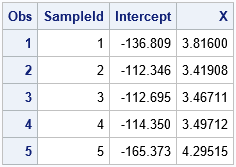The result of the analysis is a data set (PEBootResid) that contains 5,000 rows. The i_th row contains the parameter estimates for the i_th bootstrap sample. Thus, the Intercept and X columns contain the bootstrap distribution of the parameter estimates, which approximates the sampling distribution of those statistics.

### Step 4: Analyze the bootstrap distribution

The previous article includes SAS code that estimates the standard errors and covariance of the parameter estimates. It also provides SAS code for a bootstrap confidence interval for the parameters. I will not repeat those computations here. The following call to PROC SGPLOT displays a scatter plot of the bootstrap distribution of the estimates. You can see from the plot that the estimates are negatively correlated:

```/* 4. Visualize and analyze the bootstrap distribution */ title "Parameter Estimates for &NumSamples Bootstrap Samples"; title2 "Residual Resampling"; proc sgplot data=PEBootResid; label Intercept = "Estimate of Intercept" X = "Estimate of Coefficient of X"; scatter x=Intercept y=X / markerattrs=(Symbol=CircleFilled) transparency=0.7; /* Optional: draw reference lines at estimates for original data */ refline &IntEst / axis=x lineattrs=(color=blue); refline &XEst / axis=y lineattrs=(color=blue); xaxis grid; yaxis grid; run;```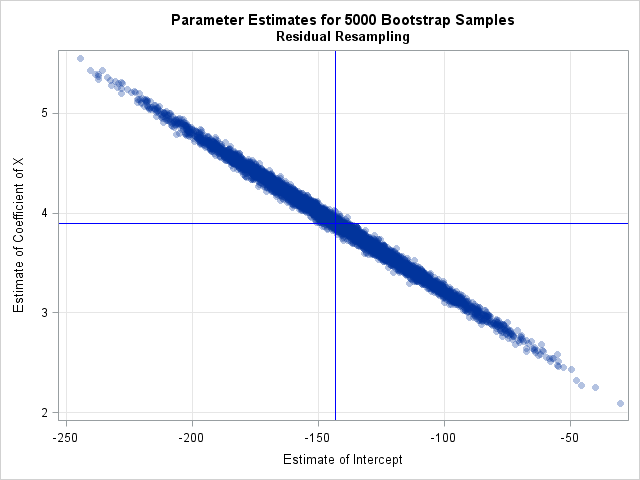### Bootstrap residuals in SAS/IML

For standard regression analyses, the previous sections show how to bootstrap residuals in a regression analysis in SAS. If you are doing a nonstandard analysis, however, you might need to perform a bootstrap analysis in the SAS/IML language. I've previously shown how to perform a bootstrap analysis (using case resampling) in SAS/IML. I've also shown how to use the SWEEP operator in SAS/IML to run thousands of regressions in a single statement. You can combine these ideas, along with an easy way to resample data (with replacement) in SAS/IML, to write a short SAS/IML program that resamples residuals, fits the samples, and plots the bootstrap estimates:

```proc iml; use RegOut; read all var {Pred X Resid}; close; /* read data */ design = j(nrow(X), 1, 1) || X; /* design matrix for explanatory variables */   /* resample residuals with replacement; form bootstrap responses */ call randseed(12345); R = sample(Resid, &NumSamples//nrow(Resid)); /* NxB matrix; each col is random residual */ YBoot = Pred + R; /* 2. NxB matrix; each col is Y_pred + resid */ M = design || YBoot; /* add intercept and X columns */ ncol = ncol(M); MpM = M`*M; /* (B+2) x (B+2) crossproduct matrix */ free R YBoot M; /* free memory */   /* Use SWEEP function to run thousands of regressions in a single call */ S = sweep(MpM, {1 2}); /* 3. sweep in intercept and X for each Y_i */ ParamEst = S[{1 2}, 3:ncol]; /* estimates for models Y_i = X */   /* 4. perform bootstrap analyses by analyzing ParamEst */ call scatter(ParamEst[1,], ParamEst[2,]) grid={x y} label={"Intercept" "X"} other="refline &IntEst /axis=x; refline &XEst /axis=y;";```

The graph is similar to the previous scatter plot and is not shown. It is remarkable how concise the SAS/IML language can be, especially compared to the procedure-based approach in Base SAS. On the other hand, the SAS/IML program uses a crossproduct matrix (MpM) that contains approximately B2 elements. If you want to analyze, say, B = 100,000 bootstrap samples, you would need to restructure the program. For example, you could analyze 10,000 samples at a time and accumulate the bootstrap estimates.

### Further thoughts and summary

The %BOOT macro also supports resampling residuals in a regression context. The documentation for the %BOOT macro contains an example. No matter how you choose to bootstrap residuals, remember that the process assumes that the model is correct and that the error terms in the model are identically distributed and independent.

Use caution if the response variable has a physical meaning, such as a physical dimension that must be positive (length, weight, ...). When you create a new response as the sum of a predicted value and a random residual, you might obtain an unrealistic result, especially if the data contain an extreme outlier. If your resamples contain a negative length or a child whose height is two meters, you might reconsider whether resampling residuals is appropriate for your data and model.

When it is appropriate, the process of resampling residuals offers a way to use the bootstrap to investigate the variance of many parameters that arise in regression. It is especially useful for data from experiments in which the explanatory variables have values that are fixed by the design.

The post Bootstrap regression estimates: Residual resampling appeared first on The DO Loop.If you want to bootstrap the parameters in a statistical regression model, you have two primary choices. The first is case resampling, which is also called resampling observations or resampling pairs. In case resampling, you create the bootstrap sample by randomly selecting observations (with replacement) from the original data. The second choice is called resampling residuals. In this method, you fit a model to the original data to obtain predicted values and residuals. You create the bootstrap sample by using the original explanatory variables but construct a new response value by adding a random residual to the predicted value. This article shows an example of case resampling in SAS. A future article shows an example of resampling residuals.

### Case resampling for bootstrapping a regression analysis

If you have some experience with bootstrapping univariate statistics, case resampling will look familiar. The main ideas behind bootstrapping are explained in the article "Compute a bootstrap confidence interval in SAS," which discusses the following steps of the bootstrap method:

1. Compute the statistic of interest for the original data
2. Resample B times from the data to form B bootstrap samples.
3. Compute the statistic on each bootstrap sample. This creates the bootstrap distribution, which approximates the sampling distribution of the statistic.
4. Use the approximate sampling distribution to obtain bootstrap estimates such as standard errors, confidence intervals, and evidence for or against the null hypothesis.

To demonstrate case resampling, consider the sampling distribution of the parameter estimates (the regression coefficients) in an ordinary least squares (OLS) regression. The sampling distribution gives insight into the variation of the estimates and how the estimates are correlated. In a case-resampling analysis, each bootstrap sample will contain randomly chosen observations from the original data. You fit the same regression model to each sample to obtain the bootstrap estimates. You can then analyze the distribution of the bootstrap estimates.

For the statistics in this article, you can compare the bootstrap estimates with estimates of standard errors and confidence intervals (CIs) that are produced by PROC REG in SAS. The procedure constructs the statistics based on several assumptions about the distribution of the errors in the OLS model. In contrast, bootstrap estimates are nonparametric and rely on fewer assumptions. Thus bootstrapping is useful when you are not sure whether the OLS assumptions hold for your data, or when you want to obtain confidence intervals for statistics (like the R-squared and RMSE) that have complicated or unknown sampling distributions.

### The statistic of interest for the original data

This example analyzes the Sashelp.Class data set, which contains data about the weights and heights of 19 students. The response variable is the Weight variable, which you can regress onto the Height variable. The regression produces two parameter estimates (Intercept and Height), standard errors, and an estimate of the "covariance of the betas." For clarity, I rename the response variable to Y and the explanatory variable to X so that each variable's role is obvious.

```/* regression bootstrap: case resampling */ data sample(keep=x y); set Sashelp.Class(rename=(Weight=Y Height=X)); /* rename to make roles easier to understand */ run;   /* 1. compute the statistics on the original data */ proc reg data=Sample plots=none; model Y = X / CLB covb; /* original estimates */ run; quit;```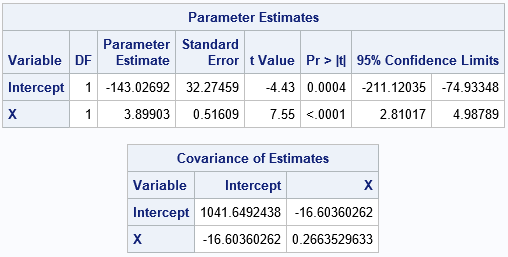The "Parameter Estimates" table includes point estimates, standard errors, and confidence intervals for the regression coefficients. The "Covariance of Estimates" table shows that the estimates are negatively correlated.

### Resample from the data

The following call to PROC SURVEYSELECT creates 5,000 bootstrap samples by sampling with replacement from the data:

```title "Bootstrap Distribution of Regression Estimates"; title2 "Case Resampling"; %let NumSamples = 5000; /* number of bootstrap resamples */ %let IntEst = -143.02692; /* original estimates for later visualization */ %let XEst = 3.89903;   /* 2. Generate many bootstrap samples by using PROC SURVEYSELECT */ proc surveyselect data=sample NOPRINT seed=1 out=BootCases(rename=(Replicate=SampleID)) method=urs /* resample with replacement */ samprate=1 /* each bootstrap sample has N observations */ /* OUTHITS use OUTHITS option to suppress the frequency var */ reps=&NumSamples; /* generate NumSamples bootstrap resamples */ run;```

The output from the SURVEYSELECT procedure is a large data set (BootCases) that contains all bootstrap samples. The SampleID variable indicates which observations belong to each sample. The NumberHits variable gives the frequency for which each of the original observations is selected. That variable should be used as a FREQ variable when analyzing the bootstrap samples. (If your procedure does not have support for a frequency variable, use the OUTHITS option on the PROC SURVEYSELECT statement to obtain an "expanded" version of the bootstrap samples.)

### Compute the bootstrap distribution

The BootCases data set contains 5,000 bootstrap samples. You can perform 5,000 regression analyses efficiently by using a BY-group analysis. You can use the BY statement in PROC REG. Be sure to use the NOPRINT option to suppress the output to the screen and use the OUTEST= option to create an output data set that contains 5,000 parameter estimates, one for each bootstrap sample.

```/* 3. Compute the statistic for each bootstrap sample */ proc reg data=BootCases outest=PEBoot noprint; by SampleID; freq NumberHits; model Y = X; run;quit;```

### Analyze the bootstrap distribution

The PEBoot data set contains 5,000 parameter estimates. To visualize the bootstrap distribution, you can create a scatter plot. The following call to PROC SGPLOT overlays reference lines that intersect at the parameter estimates for the original data.

```/* 4. Visualize bootstrap distribution */ proc sgplot data=PEBoot; label Intercept = "Estimate of Intercept" X = "Estimate of Coefficient of X"; scatter x=Intercept y=X / markerattrs=(Symbol=CircleFilled) transparency=0.7; /* Optional: draw reference lines at estimates for original data */ refline &IntEst / axis=x lineattrs=(color=blue); refline &XEst / axis=y lineattrs=(color=blue); xaxis grid; yaxis grid; run;```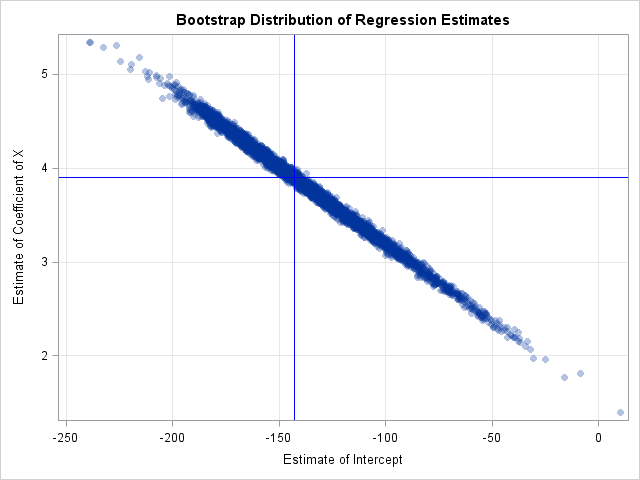The scatter plot shows that the estimates are negatively correlated, which was previously shown by the output from the COVB option on the original data. You can call PROC CORR on the bootstrap samples to obtain a bootstrap estimate of the covariance of the betas:

```proc corr data=PEBoot cov vardef=N; var Intercept X; run;```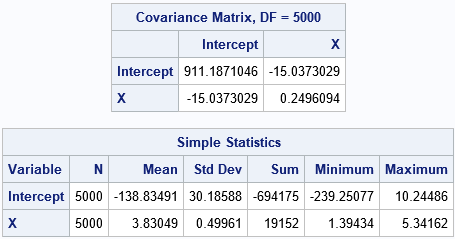The covariance matrix for the bootstrap estimates is close to the COVB matrix from PROC REG. The "Simple Statistics" table shows other bootstrap estimates. The Mean column shows the average of the bootstrap estimates; the difference between the original parameter estimates and the bootstrap average is an estimate of bias. The StdDev column estimates the standard errors of the regression estimates.

You can also use the 0.025 and 0.975 quantiles of the bootstrap estimates to construct a 95% confidence interval for the parameters. There are many ways to get the percentiles in SAS. the following statements call PROC STDIZE and print the confidence intervals for the Intercept and X variables:

```proc stdize data=PEBoot vardef=N pctlpts=2.5 97.5 PctlMtd=ORD_STAT outstat=Pctls; var Intercept X; run; proc report data=Pctls nowd; where _type_ =: 'P'; label _type_ = 'Confidence Limit'; columns ('Bootstrap Confidence Intervals (B=5000)' _ALL_); run;```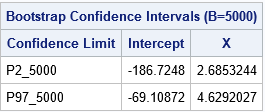The bootstrap estimates of the confidence intervals are shorter than the Wald confidence intervals from PROC REG that assume normality of errors.

### Summary

In summary, there are two primary ways to perform bootstrapping for parameters in a regression model. This article generates bootstrap samples by using "case resampling" in which observations are randomly selected (with replacement). The bootstrap process enables you to estimate the standard errors, confidence intervals, and covariance (or correlation) of the estimates. You can also use the %BOOT macro to carry out this kind of bootstrap analysis.

Case resampling is a good choice when you are modeling observational data in which the explanatory variables are observed randomly from a population. Although OLS regression treats the explanatory variable (such as Height) as a fixed effect, if you were to go into a different school and randomly select 19 students from the same grade, you would obtain a different set of heights. In that sense, the data (X and Y) can be thought of as random values from a larger population.

If you are analyzing data from a designed experiment in which the explanatory variables have a small number of specified values, you can use residual resampling, which is discussed in the next blog post.

The post Bootstrap regression estimates: Case resampling appeared first on The DO Loop.Since the late 1990s, SAS has supplied macros for basic bootstrap and jackknife analyses. This article provides an example that shows how to use the %BOOT and %BOOTCI macros. The %BOOT macro generates a bootstrap distribution and computes basic statistics about the bootstrap distribution, including estimates of bias, standard error, and a confidence interval that is suitable when the sampling distribution is normally distributed. Because bootstrap methods are often used when you do not want to assume a statistic is normally distributed, the %BOOTCI macro supports several additional confidence intervals, such as percentile-based and bias-adjusted intervals.

The %BOOT macro uses simple uniform random sampling (with replacement) or balanced bootstrap sampling to generate the bootstrap samples. It then calls a user-supplied %ANALYZE macro to compute the bootstrap distribution of your statistic.

### How to install and use the %BOOT and %BOOTCI macros

To use the macros, do the following:

1. Download the source file for the macros and save it in a directory that is accessible to SAS. For this example, I saved the source file to C:\Temp\jackboot.sas.
2. Define a macro named %ANALYZE that computes the bootstrap statistic from a bootstrap sample. The next section provides an example.
3. Call the %BOOT macro. The %BOOT macro creates three primary data sets:
• BootData is a data set view that contains B bootstrap samples of the data. For this example, I use B=5000.
• BootDist is a data set that contains the bootstrap distribution. It is created when the %BOOT macro internally calls the %ANALYZE macro on the BootData data set.
• BootStat is a data set that contains statistics about the bootstrap distribution. For example, the BootStat data set contains the mean and standard deviation of the bootstrap distribution, among other statistics.
4. If you want confidence inervals, use the %BOOTCI macro to compute up to six different interval estimates. The %BOOTCI macro creates a data set named BootCI that contains the statistics that are used to construct the confidence interval. (You can also generate multiple interval estimates by using the %ALLCI macro.)

### An example of calling the %BOOT macro

This section shows how to call the %BOOT macro. The example was previously analyzed in an article that shows how to compute a bootstrap percentile confidence interval in SAS. The statistic of interest is the skewness of the SepalWidth variable for 50 iris flowers of the species Iris virginica. The following SAS statements define the sample data and compute the skewness statistic on the original data.

```%include "C:\Temp\jackboot.sas"; /* define the %BOOT and %BOOTCI macros */   data sample(keep=x); /* data are sepal widths for 50 Iris virginica flowers */ set Sashelp.Iris(where=(Species="Virginica") rename=(SepalWidth=x)); run;   /* compute value of the statistic on original data: Skewness = 0.366 */ title 'Skewness for Petal Widths (Iris virginica)'; proc means data=sample nolabels skewness; var x; output out=SkewOut skew=Skewness; /* three output variables: _type_ _freq_ and Skewness */ run;```

The skewness statistic (not shown) is 0.366. The call to PROC MEANS is not necessary, but it shows how to create an output data set (SkewOut) that contains the Skewness statistic. By default, the %BOOT macro will analyze all numeric variables in the output data set, so the next step defines the %ANALYZE macro and uses the DROP= data set option to omit some unimportant variables that PROC MEANS automatically generates.

When you define the %ANALYZE macro, be sure to use the NOPRINT option or otherwise suppress ODS output during the bootstrap process. Include the %BYSTMT macro, which will tell the %BOOT macro to use a BY statement to efficiently implement the bootstrap analysis. The %ANALYZE macro is basically the same as the previous call to PROC MEANS, except for the addition of the NOPRINT, %BYSTMT, and DROP= options:

```%macro analyze(data=,out=); proc means noprint data=&data; %bystmt; var x; output out=&out(drop=_type_ _freq_) skew=Skewness; run; %mend;```

Although the DROP= statement is not essential, it reduces the size of the data that are read and written during the bootstrap analysis. Do NOT use a KEEP= statement in the %ANALYZE macro because the %BOOT macro will generate several other variables (called _SAMPLE_ and _OBS_) as part of the resampling process.

You can now use the %BOOT macro to generate bootstrap samples and compute basic descriptive statistics about the bootstrap distribution:

```/* creates GootData, BootDist, and BootStat data sets */ title2 'Bootstrap Analysis of Skewness'; %boot(data=sample, /* data set that contains the original data */ samples=5000, /* number of bootstrap samples */ random=12345, /* random number seed for resampling */ chart=0, /* do not display the old PROC CHART histograms */ stat=Skewness, /* list of output variables to analyze (default=_NUMERIC_) */ alpha=0.05, /* significance level for CI (default=0.05) */ print=1); /* print descriptive stats (default=1)*/   proc print data=bootstat noobs; /* or use LABEL option to get labels as column headers */ id method n; var value bootmean bias stderr biasco alcl aucl; run;```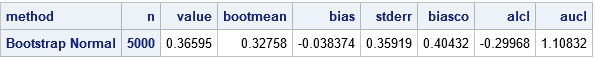I recommend that you specify the first four options. The last three options are shown in case you want to override their default values. Although the %BOOT macro prints a table of descriptive statistics, the table contains 14 columns and is very wide. To shorten the output, I used PROC PRINT to display the most important results. The table shows the estimate of the skewness statistic on the original data (VALUE), the mean of the bootstrap distribution (BOOTMEAN), the estimate for the standard error of the statistic (STDERR), and lower and upper confidence limits (ALCL and AUCL) for an approximate confidence interval under the assumption that the statistic is normally distributed. (The limits are b ± z1-α * stderr, where z1-α is the (1 - α)th normal quantile and b = value - bias is a bias-corrected estimate.)

The data for the bootstrap distribution is in the BootDist data set, so you can use PROC SGPLOT to display a histogram of the bootstrap statistics. I like to assign some of the descriptive statistics into macro variables so that I can display them on the histogram, as follows:

```/* OPTIONAL: Store bootstrap statistic in a macro variable */ proc sql noprint; select value, alcl, aucl into :Stat, :LowerCL, :UpperCL from BootStat; quit;   proc sgplot data=BootDist; /* <== this data set contains the bootstrap distribution */ histogram Skewness; refline &Stat / axis=x lineattrs=(color=red); refline &LowerCL &UpperCL / axis=x; run;```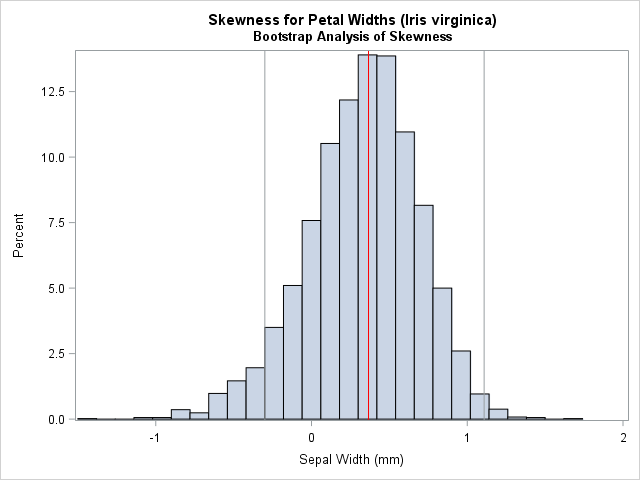### An example of calling the %BOOTCI macro

The %BOOTCI macro enables you to compute several confidence intervals (CIs) for the statistic that you are bootstrapping. The following statements display a percentile-based CI and a bias-adjusted and corrected CI.

```title2 'Percentile-Based Confidence Interval'; %bootci(PCTL); /* creates BootCI data set for Pctl CI */```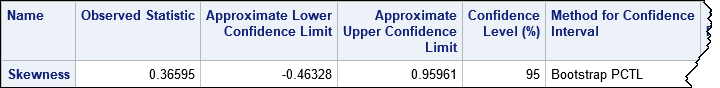The percentile-based CI is about the same width as the normal-based CI, but it is shifted to the left. The default output from the %BOOTCI macro is very wide, so sometimes I prefer to use the PRINT=0 option to suppress the output. The estimates are written to a data set named BootCI, so it is easy to use PROC PRINT to display only the statistics that you want to see, as shown in the following call that computes a bias-corrected and adjusted interval estimate:

```title2 'Bias-Adjusted and Corrected Bootstrap Confidence Interval'; %bootci(BCa, print=0); /* creates BootCI data set for BCa CI */ proc print data=BootCI noobs label; id method n; var value alcl aucl; run;```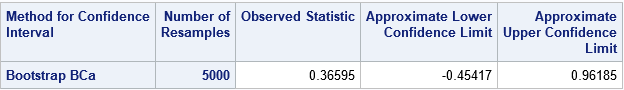Notice that each call to the %BOOTCI macro creates a data set named BootCI. In particular, the second call overwrites the data set that was created by the first call. If you want to compare the estimates, be sure to make a copy of the first BootCI data set before you overwrite it.

### The %ALLCI macro

If you want to compare multiple CIs, you can use the %ALLCI macro, which computes multiple definitions of the CIs and concatenates them into a data set named AllCI, as shown by the following:

```title2 'Comparison of Bootstrap Confidence Intervals'; %allci(print=0); proc print data=AllCI(drop=_LABEL_) noobs label; id method n; var value alcl aucl; run;```

The output (not shown) contains interval estimates for five bootstrap CIs and a jackknife CI.

Be aware the when you run the %ALLCI macro you will see several warnings in the SAS log, such as the following:

```WARNING: Variable _lo was not found on DATA file. WARNING: Variable bootmean was not found on BASE file. The variable will not be added to the BASE file.```

These warnings are coming from PROC APPEND and can be ignored. To suppress these warnings, you can edit the jackboot.sas file, search for the word 'force' on the PROC APPEND statements, and add the NOWARN option to those PROC APPEND statements. For example:
proc append data=bootci&keep base=ALLCI force nowarn; run;

### Pros and cons of using the %BOOT macro

The %BOOT, %BOOTCI, and %ALLCI macros can be a time-saver when you want to perform a basic bootstrap in SAS. However, in my opinion, they are not a substitute for understanding how to implement a bootstrap computation manually in SAS. Here are a few advantages and disadvantages of the macros:

• Advantage: The macros encapsulate the tedious steps of the bootstrap analysis.
• Advantage: The macros generate SAS data sets that you can use for additional analyses or for graphing the results.
• Advantage: The macros handle the most common sampling schemes such as simple uniform sampling (with replacement), balanced bootstrap sampling, and residual sampling in regression models.
• Advantage: The %BOOTCI macro supports many popular confidence intervals for parameters.
• Disadvantage: The macros do not provide the same flexibility as writing your own analysis. For example, the macros do not support the stratified resampling scheme that is used for a bootstrap analysis of the difference of means in a t test.
• Disadvantage: There are only a few examples of using the macros. When I first used them, I made several mistakes and had to look at the underlying source code to understand what the macros were doing.

### Summary

The %BOOT and %BOOTCI macros provide a convenient way to perform simple bootstrap analyses in SAS. The macros support several common resampling schemes and estimates for confidence intervals. Although the macros are not a replacement for understanding how to program a general, efficient, bootstrap analysis, they can be a useful tool for data analysts who want compact code to create a bootstrap analysis in SAS.

The post How to use the %BOOT and %BOOTCI macros in SAS appeared first on The DO Loop.This article shows how to implement balanced bootstrap sampling in SAS. The basic bootstrap samples with replacement from the original data (N observations) to obtain B new samples. This is called "uniform" resampling because each observation has a uniform probability of 1/N of being selected at each step of the resampling process. Within the union of the B bootstrap samples, each observation has an expected value of appearing B times.

Balanced bootstrap resampling (Davison, Hinkley, and Schechtman, 1986) is an alternative process in which each observation appears exactly B times in the union of the B bootstrap samples of size N. This has some practical benefits for estimating certain inferential statistics such as the bias and quantiles of the sampling distribution (Hall, 1990).

It is easy to implement a balanced bootstrap resampling scheme: Concatenate B copies of the data, randomly permute the B*N observations, and then use the first N observations for the first bootstrap sample, the next B for the second sample, and so forth. (Other algorithms are also possible, as discussed by Gleason, 1988). This article shows how to implement balanced bootstrap sampling in SAS.

### Balanced bootstrap samples in SAS

To illustrate the idea, consider the following data set that has N=6 observations. Five observations are clustered near x=0 and the sixth is a large outlier (x=10). The sample skewness for these data is skew=2.316 because of the influence of the outlier.

```data Sample(keep=x); input x @@; datalines; -1 -0.2 0 0.2 1 10 ;   proc means data=Sample skewness; run; %let ObsStat = 2.3163714;```

You can use the bootstrap to approximate the sampling distribution for the skewness statistic for these data. I have previously shown how to use SAS to bootstrap the skewness statistic: Use PROC SURVEYSELECT to form bootstrap samples, use PROC MEANS with a BY statement to analyze the samples, and use PROC UNIVARIATE to analyze the bootstrap distribution of skewness values. In that previous article, PROC SURVEYSELECT is used to perform uniform sampling (sampling with replacement).

It is straightforward to modify the previous program to perform balanced bootstrap sampling. The following program is based on a SAS paper by Nils Penard at PhUSE 2012. It does the following:

1. Use PROC SURVEYSEELCT to concatenate B copies of the input data.
2. Use the DATA step to generate a uniform random number for each observation.
3. Use PROC SORT to sort the data by the random values. After this step, the N*B observations are in random order.
4. Generate a variable that indicates the bootstrap sample for each observation. Alternatively, reuse the REPLICATE variable from PROC SURVEYSELECT, as shown below.
```/* balanced bootstrap computation */ proc surveyselect data=Sample out=DupData noprint reps=5000 /* duplicate data B times */ method=SRS samprate=1; /* sample w/o replacement */ run;   data Permute; set DupData; call streaminit(12345); u = rand("uniform"); /* generate a uniform random number for each obs */ run;   proc sort data=Permute; by u; run; /* sort in random order */   data BalancedBoot; merge DupData(drop=x) Permute(keep=x); /* reuse REPLICATE variable */ run;```

You can use the BalancedBoot data set to perform subsequent bootstrap analyses. If you perform a bootstrap analysis, you obtain the following approximate bootstrap distribution for the skewness statistic. The observed statistic is indicated by a red vertical line. For reference, the mean of the bootstrap distribution is indicated by a gray vertical line. You can see that the sampling distribution for this tiny data set is highly nonnormal. Many bootstrap samples that contain the outlier (exactly one-sixth of the samples in a balanced bootstrap) will have a large skewness value.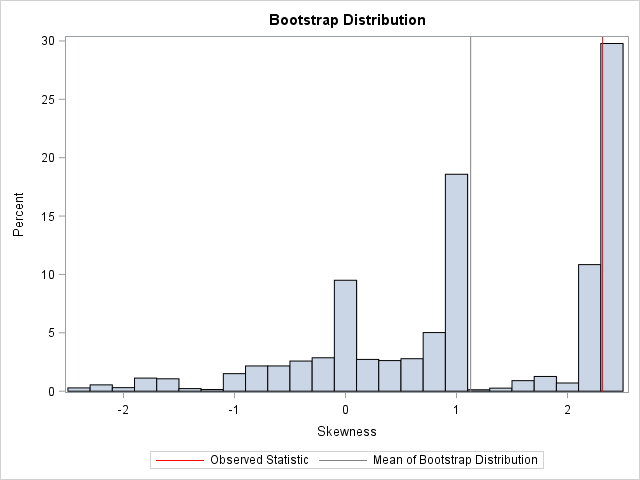To assure yourself that each of the original six observations appears exactly B times in the union of the bootstrap sample, you can run PROC FREQ, as follows:

```proc freq data=BalancedBoot; /* OPTIONAL: Show that each obs appears B times */ tables x / nocum; run;```

### Balanced bootstrap samples in SAS/IML

As shown in the article "Bootstrap estimates in SAS/IML," you can perform bootstrap computations in the SAS/IML language. For uniform sampling, the SAMPLE function samples with replacement from the original data. However, you can modify the sampling scheme to support balanced bootstrap resampling:

1. Use the REPEAT function to duplicate the data B times.
2. Use the SAMPLE function with the "WOR" option to sample without replacement. The resulting vector is a permutation of the B*N observations.
3. Use the SHAPE function to reshape the permuted data into an N x B matrix for which each column is a bootstrap sample. This form is useful for implementing vectorized computations on the columns.

The following SAS/IML program modifies the program in the previous post to perform balanced bootstrap sampling:

```/* balanced bootstrap computation in SAS/IML */ proc iml; use Sample; read all var "x"; close; call randseed(12345);   /* Return a row vector of statistics, one for each column. */ start EvalStat(M); return skewness(M); /* <== put your computation here */ finish; Est = EvalStat(x); /* 1. observed statistic for data */   /* balanced bootstrap resampling */ B = 5000; /* B = number of bootstrap samples */ allX = repeat(x, B); /* replicate the data B times */ s = sample(allX, nrow(allX), "WOR"); /* 2. sample without replacement (=permute) */ s = shape(s, nrow(x), B); /* reshape to (N x B) */   /* use the balanced bootstrap samples in subsequent computations */ bStat = T( EvalStat(s) ); /* 3. compute the statistic for each bootstrap sample */ bootEst = mean(bStat); /* 4. summarize bootstrap distrib such as mean */ bias = Est - bootEst; /* Estimate of bias */ RBal = Est || BootEst || Bias; /* combine results for printing */ print RBal[format=8.4 c={"Obs" "BootEst" "Bias"}];```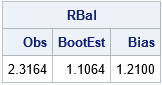As shown in the previous histogram, the bias estimate (the difference between the observed statistic and the mean of the bootstrap distribution) is sizeable.

It is worth mentioning that the SAS-supplied %BOOT macro performs balanced bootstrap sampling by default. To generate balanced bootstrap samples with the %BOOT macro, set the BALANCED=1 option, as follows:
%boot(data=Sample, samples=5000, balanced=1) /* or omit BALANCED= option */
If you want uniform (unbalanced) samples, call the macro as follows:
%boot(data=Sample, samples=5000, balanced=0).

In conclusion, it is easy to generate balanced bootstrap samples. Balanced sampling can improve the efficiency of certain bootstrap estimates and inferences. For details, see the previous references of Appendix II of Hall (1992).

The post Balanced bootstrap resampling in SAS appeared first on The DO Loop.A previous article provides an example of using the BOOTSTRAP statement in PROC TTEST to compute bootstrap estimates of statistics in a two-sample t test. The BOOTSTRAP statement is new in SAS/STAT 14.3 (SAS 9.4M5). However, you can perform the same bootstrap analysis in earlier releases of SAS by using procedures in Base SAS and SAS/STAT. This article gives an example of how to bootstrap in SAS.

### The main steps of the bootstrap method in SAS

A previous article describes how to construct a bootstrap confidence interval in SAS. The major steps of a bootstrap analysis follow:

1. Compute the statistic of interest for the original data
2. Resample B times (with replacement) from the data to form B bootstrap samples. The resampling process should respect the structure of the analysis and the null hypothesis. In SAS it is most efficient to use the DATA step or PROC SURVEYSELECT to put all B random bootstrap samples into a single data set.
3. Use BY-group processing to compute the statistic of interest on each bootstrap sample. The BY-group approach is much faster than using macro loops. The union of the statistic is the bootstrap distribution, which approximates the sampling distribution of the statistic under the null hypothesis.
4. Use the bootstrap distribution to obtain bootstrap estimates of bias, standard errors, and confidence intervals.

### Compute the statistic of interest

This article uses the same bootstrap example as the previous article. The following SAS DATA step subsets the Sashelp.Cars data to create a data set that contains two groups: SUV" and "Sedan". There are 60 SUVs and 262 sedans. The statistic of interest is the difference of means between the two groups. A call to PROC TTEST computes the difference between group means for the data:

```data Sample; /* create the sample data. The two groups are "SUV" and "Sedan" */ set Sashelp.Cars(keep=Type MPG_City); if Type in ('Sedan' 'SUV'); run;   /* 1. Compute statistic (difference of means) for data */ proc ttest data=Sample; class Type; var MPG_City; ods output Statistics=SampleStats; /* save statistic in SAS data set */ run;   /* 1b. OPTIONAL: Store sample statistic in a macro variable for later use */ proc sql noprint; select Mean into :Statistic from SampleStats where Method="Satterthwaite"; quit; %put &=Statistic;```
`STATISTIC= -4.9840`

The point estimate for the difference of means between groups is -4.98. The TTEST procedure produces a graph (not shown) that indicates that the MPG_City variable is moderately skewed for the "Sedan" group. Therefore you might question the usefulness of the classical parametric estimates for the standard error and confidence interval for the difference of means. The following bootstrap analysis provides a nonparametric estimate about the accuracy of the difference of means.

### Resample from the data

For many resampling schemes, PROC SURVEYSELECT is the simplest way to generate bootstrap samples. The documentation for PROC TTEST states, "In a bootstrap for a two-sample design, random draws of size n1 and n2 are taken with replacement from the first and second groups, respectively, and combined to produce a single bootstrap sample." One way to carry out this sampling scheme is to use the STRATA statement in PROC SURVEYSELECT to sample (with replacement) from the "SUV" and "Sedan" groups. To perform stratified sampling, sort the data by the STRATA variable. The following statements sort the data and generate 10,000 bootstrap samples by drawing random samples (with replacement) from each group:

```/* 2. Sample with replacement from each stratum. First sort by the STRATA variable. */ proc sort data=Sample; by Type; run;   /* Then perform stratified sampling with replacement */ proc surveyselect data=Sample out=BootSamples noprint seed=123 method=urs /* with replacement */ /* OUTHITS */ /* use OUTHITS option when you do not want a frequency variable */ samprate=1 reps=10000; /* 10,000 resamples */ strata Type; /* sample N1 from first group and N2 from second */ run;```

The BootSamples data set contains 10,000 random resamples. Each sample contains 60 SUVs and 262 sedans, just like the original data. The BootSamples data contains a variable named NumberHits that contains the frequency with which each original observation appears in the resample. If you prefer to use duplicated observations, specify the OUTHITS option in the PROC SURVEYSELECT statement. The different samples are identified by the values of the Replicate variable.

### BY-group analysis of bootstrap samples

Recall that a BY-group analysis is an efficient way to process 10,000 bootstrap samples. Recall also that it is efficient to suppress output when you perform a large BY-group analysis. The following macros encapsulate the commands that suppress ODS objects prior to a simulation or bootstrap analysis and then permit the objects to appear after the analysis is complete:

```/* Define useful macros */ %macro ODSOff(); /* Call prior to BY-group processing */ ods graphics off; ods exclude all; ods noresults; %mend;   %macro ODSOn(); /* Call after BY-group processing */ ods graphics on; ods exclude none; ods results; %mend;```

With these definitions, the following call to PROC TTEST computes the Satterthwaite test statistic for each bootstrap sample. Notice that you need to sort the data by the Replicate variable because the BootSamples data are ordered by the values of the Type variable. Note also that the NumberHits variable is used as a FREQ variable.

```/* 3. Compute statistics */ proc sort data = BootSamples; by Replicate Type; run;   %ODSOff /* suppress output */ proc ttest data=BootSamples; by Replicate; class Type; var MPG_City; freq NumberHits; /* Use FREQ variable in analysis (or use OUTHITS option) */ ods output ConfLimits=BootDist(where=(method="Satterthwaite") keep=Replicate Variable Class Method Mean rename=(Mean=DiffMeans)); run; %ODSOn /* enable output */```

### Obtain estimates from the bootstrap distribution

At this point in the bootstrap example, the data set BootDist contains the bootstrap distribution in the variable DiffMeans. You can use this variable to compute various bootstrap statistics. For example, the bootstrap estimate of the standard error is the standard deviation of the DiffMeans variable. The estimate of bias is the difference between the mean of the bootstrap estimates and the original statistic. The percentiles of the DiffMeans variable can be used to construct a confidence interval. (Or you can use a different interval estimate, such as the bias-adjusted and corrected interval.) You might also want to graph the bootstrap distribution. The following statements use PROC UNIVARIATE to compute these estimates:

```/* 4. Plot sampling distribution of difference of sample means. Write stats to BootStats data set */ proc univariate data=BootDist; /* use NOPRINT option to suppress output and graphs */ var DiffMeans; histogram DiffMeans; /* OPTIONAL */ output out=BootStats pctlpts =2.5 97.5 pctlname=P025 P975 pctlpre =Mean_ mean=BootMean std=BootStdErr; run;   /* use original sample statistic to compute bias */ data BootStats; set BootStats; Bias = BootMean - &Statistic; label Mean_P025="Lower 95% CL" Mean_P975="Upper 95% CL"; run;   proc print data=BootStats noobs; var BootMean BootStdErr Bias Mean_P025 Mean_P975; run;```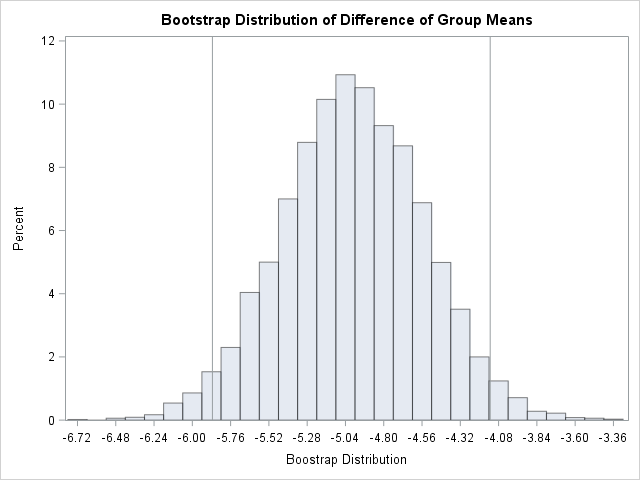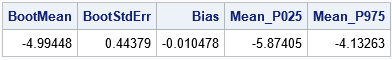The results are shown. The bootstrap distribution appears to be normally distributed. This indicates that the bootstrap estimates will probably be similar to the classical parametric estimates. For this problem, the classical estimate of the standard error is 0.448 and a 95% confidence interval for the difference of means is [-5.87, -4.10]. In comparison, the bootstrap estimates are 0.444 and [-5.87, -4.13]. In spite of the skewness of the MPG_City variable for the "Sedan" group, the two-sample Satterthwaite t provides similar estimates regarding the accuracy of the point estimate for the difference of means. The bootstrap statistics also are similar to the statistics that you can obtain by using the BOOTSTRAP statement in PROC TTEST in SAS/STAT 14.3.

In summary, you can use Base SAS and SAS/STAT procedures to compute a bootstrap analysis of a two-sample t test. Although the "manual" bootstrap requires more programming effort than using the BOOTSTRAP statement in PROC TTEST, the example in this article generalizes to other statistics for which a built-in bootstrap option is not supported. This article also shows how to use PROC SURVEYSELECT to perform stratified sampling as part of a bootstrap analysis that involves sampling from multiple groups.

The post The bootstrap method in SAS: A t test example appeared first on The DO Loop.